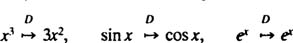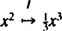## Linear Transformations

If you are faced with an IVP that involves a linear differential equation with constant coefficients, you can proceed by the method of undetermined coefficients or variation of parameters and then apply the initial conditions to evaluate the constants. However, what if the nonhomogeneous right‐hand term is discontinuous? There exists a method for solving such problems that can also be used to solve less frightening IVP's (that is, ones that do not involve discontinuous terms) and even some equations whose coefficients are not constants. One of the features of this alternative method for solving IVP's is that the values of the parameters are not found after the general solution has been obtained. Instead, the initial conditions are incorporated right into the initial stages of the solution, so when the final step is completed, the arbitrary constants have already been evaluated.

A function is usually introduced as a rule which acts on a number to produce a unique numerical result. That is, a function accepts a number as input and produces a number as output. For instance, consider the function defined by the equation f( x) = x 2; it specifies a particular operation to be performed on any given value of x. When this function acts on the number 3, for example, it gives the result 9, a fact which can be symbolized as follows:However, functions are not restricted to acting only on numbers to produce other numbers. A function can also act on a function to produce another function. These “superfunctions” are often referred to as operators or transformations. Therefore, an operator accepts a function as input and produces a function as output.

A familiar example is the differentiation operator, D:This operator acts on a (differentiable) function to produce another function: namely, the derivative of the input function. For example,Another well‐known example is the integration operator, which acts on an (integrable) function to produce another function: its integral. Since an operator is a function, it must produce one and only one output for each input; therefore, it makes sense here to consider an integration operator I of the form[The letter t, a dummy variable, is chosen simply to distinguish it from x, the upper limit of integration. It's usually considered bad form to write, for example, ∫ 0 x f( x) dx.]

To illustrate, if f( x) = x 2, thenthat is,Both of these operators, differentiation D and integration I, enjoy an important property known as linearity. Any operator or transformation T is said to be linear if both of the following conditions,always hold for all constants c and all admissible functions f and g.

Since

• the derivative of a constant multiple of a function is equal to the constant times the derivative of the function,

and since

• the derivative of the sum of two functions is the sum of the derivatives,

differentiation is indeed linear. Furthermore, since both of the previous statements remain true when “derivative” is replaced by “integral,” integration is also a linear transformation.